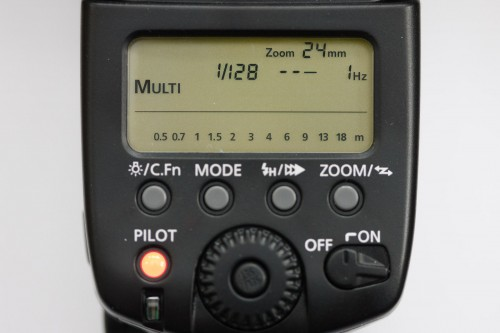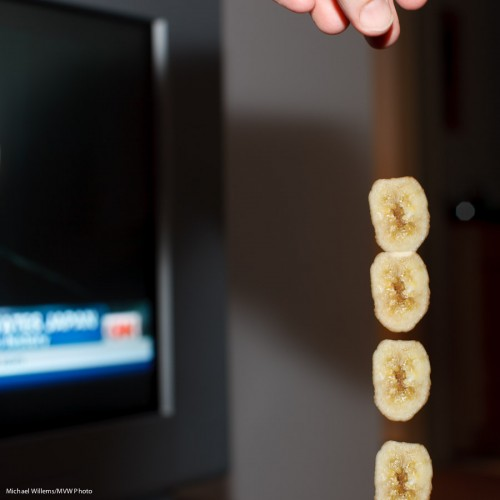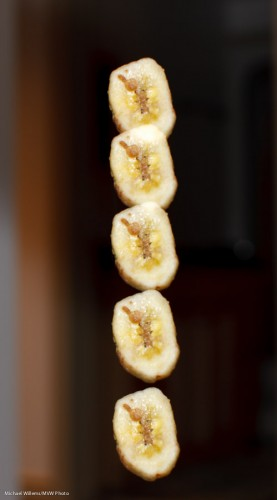# Do try this at home

Did you know that if you have a modern sophisticated flash like a Canon 580 EX, you can set it to strobe/repeated flash mode (“Multi”)?

This makes the flash fire a number of subsequent pulses, at a certain frequency, at a certain power level.To activate this mode on a 580EX, Press the MODE button until MULTI appears; then set power level, number of pulses, and frequency in pulses per second.

Which then gives you pictures like this, of my hand dropping a slice of dried banana:And another version:Those were taken with the flash set to fire 10 pulses at 50 Hz, each at 1/32nd of full power. The camera was in manual mode.

(You do not even have to meter: look at the back: the flash indicates how far away the subject should be with your chosen flash/aperture and ISO settings!)

Challenge to physics students out there: use this image to estimate the acceleration of gravity, in meters per second squared. Or, if you already know that it is 9.81 meters per second squared, use this to work out the size of the banana)?

This (the flash part, not the physics part!) is among the many subjects I teach at my Advanced workshops, like the David Honl special on Saturday in Toronto., for which I believe there are just a couple of spots left.

## 4 thoughts on “Do try this at home”

1.Deborah on said:

This is a terrific physics experiment. You could position a ruler right beside the banana and so include the scale right in the photo…then see how close the acceleration comes to 9.8 m/s^2. Then figure out if air resistance really matters for a falling banana. It would be fun too to start the fall from several meters above the camera and then from only a few centimeters above and compare the two. No end to the ways a camera is a great tool for teaching physics!

• Exactly. Since you can set frequency of the flash you can set it to match the scale at both ends. I bet you would find 9.81 m/s^2 since air resistance at these distances and speeds (and for this flat shape, falling straight) is likely to be negligible. And you could figure how how much faster a can of lead falls than a can of feathers! (Remember, they did this on the moon? Feather and lead? Well have you ever. Same acceleration)

2.Leaman c on said:

Michael, let me have a crack at this:

Assumptions:
1. The diameter of the banana is 4cm (which should be fairly accurate).
2. In your photo, I can see that with each successive photo from where the banana overlaps less and less, is approximately 10% of the diameter. (First overlaps by 20%, second by 10%, next 0%, then +10%, etc)

Analysis:
1. Based on assumption 1) and 2) I can deduce that there were 8 additional positions (for a total of 12 positions) that were not captured from the point you let go of the banana to when you hit the shutter for the first time. Therefore from time t=0, the distance travelled is the summation 4cm x 10% +4cm x 20% + 4cm x 30% …. etc. Using this logic, the total distance travelled from when you let go to the last picture taken is 0.264m.

2. The total time of travel for the banana is determined by the flash cycle of 50Hz. We know that there are a total of 12 positions of the banana which could have been frozen by the flash at that rate. therefore the total travel time is 12/50 =0.24 s

3. Using the information calculated in 1 and 2, and any of the kinematic equations:
d=v1 x t + 0.5at^2
v1=0
solve for a.
and based on the assumptions 1) and 2).:
a=9.17m/s^2

pretty close!
Unless my logic is flawed somewhere.

• I think your logic is correct, and that number is very close.